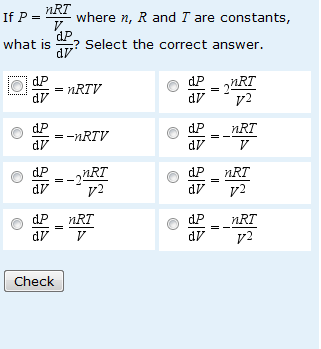# Is the difficulty calculus or negative numbers?

As you’ll have realised, I’m currently analysing responses to questions on calculus. Maths for Science teaches just very basic differentiation and the position of the chapter on differentiation will be different in the revised edition of the course. In the new edition, the chapter will be where it belongs in a logical story line, immediately after the chapter on graphs and gradient. In the old edition, we thought differentiation might be a step too far for some students so offered Differentiation (Chapter 10) as a choice with Statistical Hypothesis Testing (Chapter 9). Add in the fact that we still allowed students to answer questions on both chapters if they wanted to, and the fact that many of the Chapter 10 questions are multiple choice, and it is really quite difficult to tell whether students are guessing answers to the Chapter 10 questions – even the summative ones. This is characteristically different from behaviour on earlier questions where it is very obvious that most students are not guessing.

An extension of this is that it is quite difficult to assess what the real difficulties are that students experience with the chapter on differentiation. We have some familiar stories e.g. answers where a function has been differentiated correctly and values subsituted correctly, but then answers have been given to an inappropriate number of significant figures. Hey ho – I think we get too hung up about this.

Now compare the two questions given below.For the question on the left-hand side (where the correct answer is negative)  53.6% of responses are correct and only 8.9% give the answer that is positive but otherwise correct.For the question on the right-hand side (where the expression to be differentiated is negative so the correct answer is positive) only 39.9% of responses are correct and 17.3% give the answer that is negative but otherwise correct. So students are either failing to see the negative sign in the question or they are becoming confused by the double negation.

I suppose I should be pleased that students can do the differentiation, but I’m depressed that we just seem to gather more evidence of students falling over little details.

This entry was posted in calculus, derivative, mathematical misunderstandings, negative numbers, question analysis and tagged , , , . Bookmark the permalink.